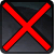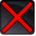# [east_obj_area]Conditions

## Related effects, buffs and debuffs

• [hidden] [does not expire]Effect #1

 Slot: Buff Duration: passive Tick rate: 30 Sek. # occurrences: 0 Tags: tag.​abl.​pvp.​badge_volume
• On Apply

Perform the following actions:

• Custom
- Param 2 = (string)
- Param 3 = (string)
- Param 4 = (string)
- Param 5 = (string)
- Param 6 = (string)
- Param 7 = (string)
- Param 8 = (string)
- Param 9 = (string)
- Param 1 = (int) 726600482482484207
• Just before this effect is removed

Perform the following actions:

• Custom
- Param 2 = (string)
- Param 3 = (string)
- Param 4 = (string)
- Param 5 = (string)
- Param 6 = (string)
- Param 7 = (string)
- Param 8 = (string)
- Param 9 = (string)
- Param 1 = (int) 6464478966400623586
• When effect ticks

Perform the following actions:

• Execute Hydra Hook
- Event Name = (string) objective_trickle_east
- Object Self = (int) 1